# High School Math : Understanding Coterminal Angles

## Example Questions

### Example Question #1 : Understanding Coterminal Angles

Which of the following angles is coterminal with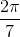?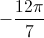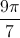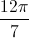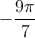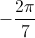Explanation:

For an angle to be coterminal with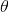, that angle must be of the form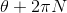for some integer- or, equivalently, the difference of the angle measures multiplied by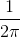must be an integer. We apply this test to all five choices.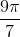: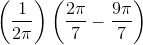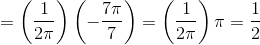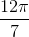: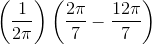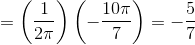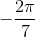: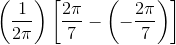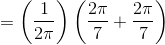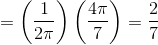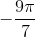: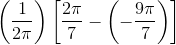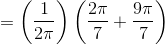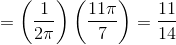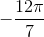: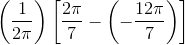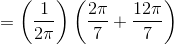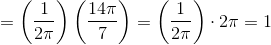is the correct choice, since only that choice passes our test.

### Example Question #1 : Understanding Coterminal Angles

Find a coterminal angle for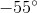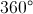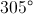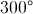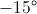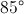Explanation:

Coterminal angles are angles that, when drawn in the standard position, share a terminal side. You can find these angles by adding or subtracting 360 to the given angle. Thus, the only angle measurement that works from the answers given is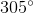### Example Question #2 : Understanding Coterminal Angles

Which of the following angles is coterminal with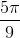?

Each angle given in the other choices is coterminal with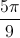.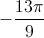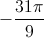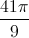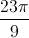Each angle given in the other choices is coterminal with.

Explanation:

For an angle to be coterminal with, that angle must be of the formfor some integer- or, equivalently, the difference of the angle measures multiplied bymust be an integer. We apply this test to all four choices.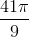: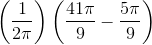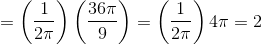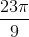: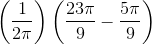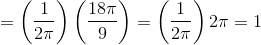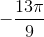: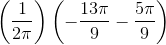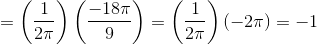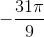: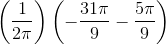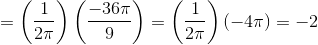All four choices pass the test, so all four angles are coterminal with.

### Example Question #4 : Understanding Coterminal Angles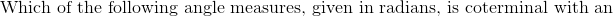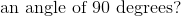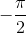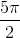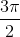Explanation: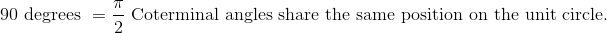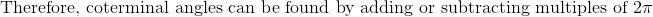.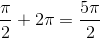### Example Question #5 : Understanding Coterminal Angles

Which of the following choices represents a pair of coterminal angles?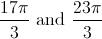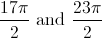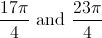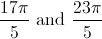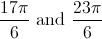Explanation:

For two angles to be coterminal, they must differ by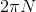for some integer- or, equivalently, the difference of the angle measures multiplied bymust be an integer. We apply this test to all five choices.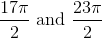: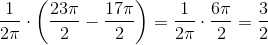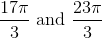: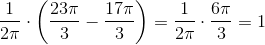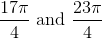: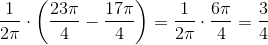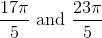: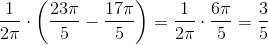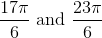: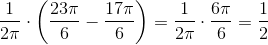The only angles that pass the test - and are therefore coterminal - are.